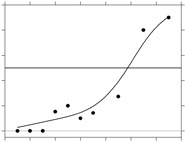# Model-free estimation of a psychometric function

## Functions

`bandwidth_bootstrap`

Bootstrap estimate of the optimal bandwidth `h` for a local polynomial estimate of the psychometric function with specified guessing and lapsing rates.

`bandwidth_cross_validation`

Cross-validation bandwidth for a local polynomial estimate of the psychometric function with specified guessing and lapsing rates.

`bandwidth_optimal`

Optimal bandwidth for a local polynomial estimate of the psychometric function with specified guessing and lapsing rates. The difference between this function and `bandwidth_cross_validation` is that here the true psychometric function is known.

`bandwidth_plugin`

AMISE optimal bandwidth for a local polynomial estimate of the psychometric function.

`binom_lims`

Maximum likelihood estimates of the parameters of the psychometric function with guessing and lapsing rates, only guessing rate, or only lapsing rate.

`binom_revweib`

Maximum likelihood estimates of the parameters of the reverse Weibull model for the psychometric function.

`binom_weib`

Maximum likelihood estimates of the parameters of the Weibull model for the psychometric function.

`binomfit_lims`

Maximum likelihood estimates of the parameters in a binomial generalised linear model with guessing and lapsing rates.

`binomval_lims`

Fitted values at points `xfit` for a binomial generalized linear model with coefficients `b` and guessing and lapsing rates.

`bootstrap_ci_sl`

Bootstrap estimate of a confidence interval at a significance level alpha for the estimated slope for the local polynomial estimate of the psychometric function with guessing and lapsing rates. The confidence interval is based on bootstrap percentiles.

`bootstrap_ci_th`

Bootstrap estimate of a confidence interval at a significance level alpha for the estimated threshold for the local polynomial estimate of the psychometric function with guessing and lapsing rates. The confidence interval is based on bootstrap percentiles.

`bootstrap_sd_sl`

Bootstrap estimate of the standard deviation of the estimated slope for the local polynomial estimate of the psychometric function with guessing and lapsing rates.

`bootstrap_sd_th`

Bootstrap estimate of the standard deviation of the estimated threshold for the local polynomial estimate of the psychometric function with guessing and lapsing rates.

`comploglog_link`

Complementary log-log link in cell form for use with `GLMFIT`, `GLMVAL` and other Matlab GLM functions. The guessing rate and lapsing rate are fixed; hence `link` is a function of only one variable.

`deviance`

Deviance for the fitted values of the psychometric function `pfit`.

`locglmfit`

Local polynomial estimator for the psychometric function and `eta` function (psychometric function transformed by `link`) for binomial data; also returns the hat matrix `H`.

`logit_link`

Logit link in cell form for use with `GLMFIT`, `GLMVAL` and other Matlab GLM functions. The guessing rate and lapsing rate are fixed; hence `link` is a function of only one variable.

`loglog_link`

Log-log link in cell form for use with `GLMFIT`, `GLMVAL` and other Matlab GLM functions. The guessing rate and lapsing rate are fixed; hence `link` is a function of only one variable.

`probit_link`

Probit link in cell form for use with `GLMFIT`, `GLMVAL` and other Matlab GLM functions. The guessing rate and lapsing rate are fixed; hence `link` is a function of only one variable.

`revweibull_link`

Reverse Weibull link in cell form for use with `GLMFIT`, `GLMVAL` and other Matlab GLM functions. The guessing rate and lapsing rate are fixed, and the power parameter is set to be equal `K`; hence `link` is a function of only one variable.

`threshold_slope`

Approximate value of `x` (`=x_th`) for which the value of the estimated psychometric function is equal to `thresh` and the approximate value of slope at `x_th`.

`weibull_link`

Weibull link in cell form for use with `GLMFIT`, `GLMVAL` and other Matlab GLM functions. The guessing rate and lapsing rate are fixed, and the power parameter is set to be equal `K`; hence `link` is a function of only one variable.